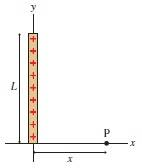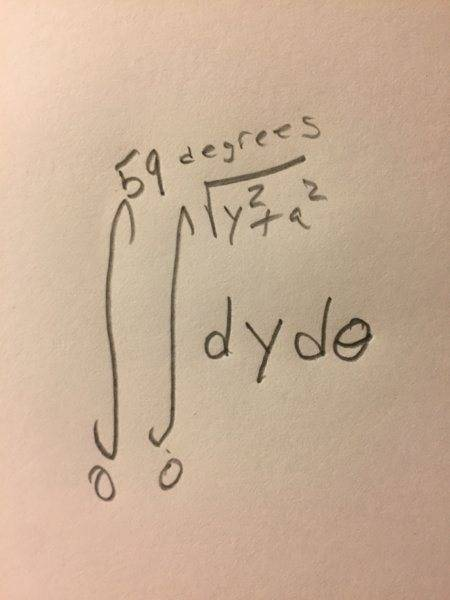# Find the Y component of Electric field at a point

## Homework Statement

The figure below shows a thin, vertical rod of length L with total charge Q. The indicated point P is a horizontal distance x from the one end of the rod. What is the electric field at point P. Express your answer in component notation in the two blanks below.

L = 5.0 cm, Q = 3.0 nC, and x = 3.0 cm.kq/r^2

## The Attempt at a Solution

I found the x component to be 15000N/C

theta = arctan(5/3) = 59 deg
total charge = [(8.99x10^9)(3x10^-9)]/((0.03)^2) = 29966
x component = 29966cos59=15417 with sig figs is 15000

so now the y component should just be 29966sin59 = 25685 rounded to 26000 N/C but that doesn't make sense because the y component should be facing down

#### Attachments

even when I did theta = -59 degrees the answer was incorect

haruspex
Homework Helper
Gold Member
total charge = [(8.99x10^9)(3x10^-9)]/((0.03)^2) = 29966
Not all the charge is at distance 3cm from P.

•isukatphysics69
Not all the charge is at distance 3cm from P.
So did I just get lucky with the first answer??

im confused

Ok I think I see what youre saying. I got the distance from the top of the rod as
[(8.99x10^9)(3x10^-9)]/((0.058^2))=7932
7932sin59 = 6800

incorrect

@haruspex

I tried generalizing from the middle

[(8.99x10^9)(3x10^-9)]/((0.039^2))=17685
(new angle arctan(2.5/3) = 39 deg
17685sin(39) = 11321

incorrect

haruspex
Homework Helper
Gold Member
Ok I think I see what youre saying. I got the distance from the top of the rod as
[(8.99x10^9)(3x10^-9)]/((0.058^2))=7932
7932sin59 = 6800

incorrect

@haruspex
Consider an element length dy at distance y up the rod. What is the charge on it? What field does it generate at P? What is the x component of that?

Consider an element length dy at distance y up the rod. What is the charge on it? What field does it generate at P? What is the x component of that?
so the charge on dy is kq/r^2 at point P the x component is (kq/r^2)cos(theta)

my x component is correct tho, I thought the y should be simple just sin of the angle @haruspex

haruspex
Homework Helper
Gold Member
so the charge on dy is kq/r^2
That s not a charge.
What is the charge on a section of length dy?
If it is distance y up the rod, how far is it from point P?

Last edited:
•isukatphysics69
That s not a charge.
What is the charge on a section of length dy?
Of it is distance y up the rod, how far is it from point P?
would it be dy/3? im really not understanding. dy is sqrt(y^2+9) away

haruspex
Homework Helper
Gold Member
would it be dy/3?
No.
What is the charge density along the rod? If the whole rod length L carries charge Q, how much charge is there on section length dy?
dy is sqrt(y^2+9) away
Units! But better still, don't plug in numbers yet, just call it x2, not 9.

•isukatphysics69
No.
What is the charge density along the rod? If the whole rod length L carries charge Q, how much charge is there on section length dy?
Is it Q*dy????

Help?

GOT IT!!!!!!!!!!!!!!!!!!!!!!!!!!!!!!!!!!!!!!!!!!!!!!!!!!!!!!!!!!!!!!!!!!!!!!!!!!!!!!!!!!!!!!!!!!!!!!!!!!!!!!!!!!!!!!!!!!!!!!!!!!!!!!!!!! >=[

haruspex
Homework Helper
Gold Member
Is it Q*dy????
The charge Q is spread out along a rod length L, what is the charge density (charge per unit length)?

•isukatphysics69
The charge Q is spread out along a rod length L, what is the charge density (charge per unit length)?
Then I think it is dQ*dL

I got the answer partial correct 1.8/2 points with the answer -7900N/C

Im confused why im getting partial credit

[(8.99x10^9)(3x10^-9)]/((sqrt(.05^2+.03^2)^2) = 7932 taking the negative because +/- sqrt = -7932

1.8/2 points

SammyS
Staff Emeritus
Homework Helper
Gold Member
Is it Q*dy????
You seem to be guessing, rather than answering the specific question haruspex asked you.

To ask basically the same thing:
If the length of the rod is 5.0 cm, and the charge on the rod is 3.0 nC, how much charge is on each 1 cm of the rod?
How would you write that using symbols: L for length and Q for the charge?

SammyS
Staff Emeritus
Homework Helper
Gold Member
Im confused why im getting partial credit

[(8.99x10^9)(3x10^-9)]/((sqrt(.05^2+.03^2)^2) = 7932 taking the negative because +/- sqrt = -7932

1.8/2 points
Slow down. You're nowhere near ready to solve this. The solution will require that you use Calculus to do integration.

Slow down. You're nowhere near ready to solve this. The solution will require that you use Calculus to do integration.
Oh god.. I have to go to physics class right now. im getting frustrated awith this anyway I have to step away

SammyS
Staff Emeritus
Homework Helper
Gold Member
So did I just get lucky with the first answer??
You mean to ask if you got lucky with getting the correct numerical answer for the x component of the leectric field (which is in your "other" thread.)

The answer is absolutely yes, you got lucky.

You used cosine, when what was required there was to use sine.

Both components require the use of integral calculus.

Last edited:
hey @SammyS do I have to use trig substitution?

SammyS
Staff Emeritus
Homework Helper
Gold Member
hey @SammyS do I have to use trig substitution?
Most likely you will.

You will first have to come up with a linear charge density and will need to set up an integral.

Most likely you will.

You will first have to come up with a linear charge density and will need to set up an integral.
omfg I hate doing trig sub thought I was done with that after calc 2 im really pissed right now

im just going to sleep

linear algebra test tomorrow and 2 programming assignments due theres no way ill get this frikkin degree I don't even have a job right now and I cant even keep up with the work

Im thinking I can use a double integral so that I don't have to do the frikking trig substitution.. plus I took calc 3 in the summer so I should be using double integralsdoes this look good?

#### Attachments

SammyS
Staff Emeritus
Homework Helper
Gold Member
Im thinking I can use a double integral so that I don't have to do the frikking trig substitution.. plus I took calc 3 in the summer so I should be using double integrals
[ ATTACH=full]230927[/ATTACH]

does this look good?
I can't imagine how a double integral can even be used here. Don't even be concerned about Trig substitution at this point in solving the problem. That integral doesn't correspond to this problem at all.

Let me remind you of the advice haruspex gave you in post #8
Consider an element of charge with length dy at distance y up the rod. What is the charge on it? What field does it generate at P? What is the x component of that?
To use this idea, you will need to use the linear charge density, usually represented by the variable λ. You can get a value for λ from the given information, but there's no need bother with that yet.

( By the way: It looks like you will not need to use Trig. substitution for finding the y-component. )

Last edited:
I just did the integration and got -140677.0588 and that was incorrect.
I had my integral limits from 0 to 0.05 and ∫(kqy(dy)/(a(x^2+y^2)3/2))

used U substitution and was a pretty straight forward integral. not sure what I did wrong here

SammyS
Staff Emeritus
Homework Helper
Gold Member
I just did the integration and got -140677.0588 and that was incorrect.
I had my integral limits from 0 to 0.05 and ∫(kqy(dy)/(a(x^2+y^2)3/2))

used U substitution and was a pretty straight forward integral. not sure what I did wrong here
It's much more helpful to do the integration using symbols. Plug in the numerical values later. There is virtually no way for anyone to look at the result, −140677.0588 and tell what might be wrong with it. It doesn't even have units.

That is pretty close to being the correct integral.
Having ##\ q\ ## there isn't correct. That should be ##\ \lambda\ ##, the linear charge density.
What is ' a ' ? (It's in the denominator.)
For integration limits, 0 to L .​

What do you get for the integral, prior to substituting the integration limits?.

•isukatphysics69
It's much more helpful to do the integration using symbols. Plug in the numerical values later. There is virtually no way for anyone to look at the result, −140677.0588 and tell what might be wrong with it. It doesn't even have units.

That is pretty close to being the correct integral.
Having ##\ q\ ## there isn't correct. That should be ##\ \lambda\ ##, the linear charge density.
What is ' a ' ? (It's in the denominator.)
For integration limits, 0 to L .​

What do you get for the integral, prior to substituting the integration limits?.
I had 'a' as the length of the entire rod, I will try again

This is really frustrating but im staying calm like a monk

SammyS
Staff Emeritus
Homework Helper
Gold Member
I had 'a' as the length of the entire rod, I will try again
Well in that case, that does give you a factor of q/L, which is correct and it's needed because, q/L is λ .

Making that replacement, makes your integral:
∫(kqy(dy)/(L(x2+y2)3/2)) with limits from 0 to L .

In more readable form, using LaTeX that can be written:
##\displaystyle \frac{kq}{L} \int_0^L \frac {y\,dy}{(x^2+y^2)^{3/2}} \,,\ ## where I factored out the constant factors.​
.